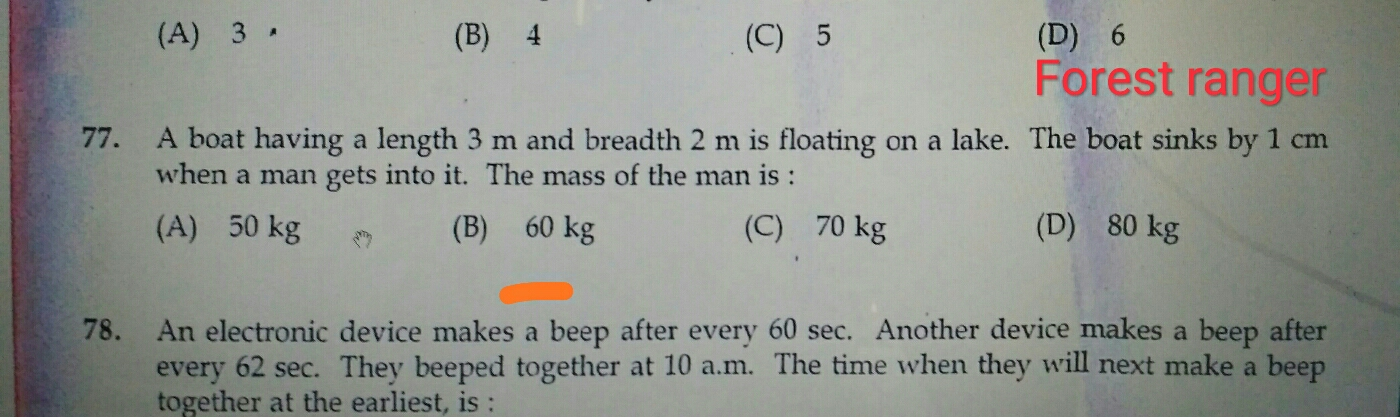H
Hari
22 Feb 19

Ithenganeyanu cheyyuka (QN:77)## Replies to this post

S
Sooraj

Calculate Volume of water displaces then will multiply with the density of water.

Volume of water dispalced = 3×2×0.01 = 0.06 m³

Mass of man =Volume of water displaced × Density of water

``````    = 0.06 × 1000 = 60 kg
``````
0